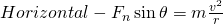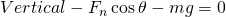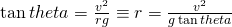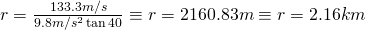# Radius of a circle in relation to banking angle

A question was posed in Physics as follows:

An airplane is flying in a horizontal circle at a speed of 480 km/hr. If its wings are titled at a 40° angle relative to the horizontal, what is the radius of the circle (in km) in which the plane is flying? Assume that the required force is proved by the lift which acts perpendicular to the wing surface.

Now let’s identify the information that you need to know:

Velocity = 480 km/hr (133.3 m/s) (you need to convert this for the calculations to work right)
Angle = 40 degrees
Gravitational Acceleration = 9.8 m/s^2

What is important to note in this problem is that the force is modified  by the 40 degree angle meaning that the circle can be tighter because the force is higher.  The easiest way to do this is to use a derived formula.  I’m going to shorten it a bit for time sake but you should be aware that it comes from this combination of horizontal and vertical forces:This relationship gives us the following formula:Now we apply this to the known values to get our turning radius:As always, please feel free to comment and or correct any conceptual or mathematical errors on my part.  Thanks!